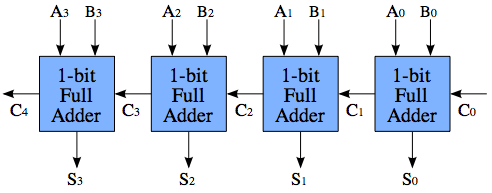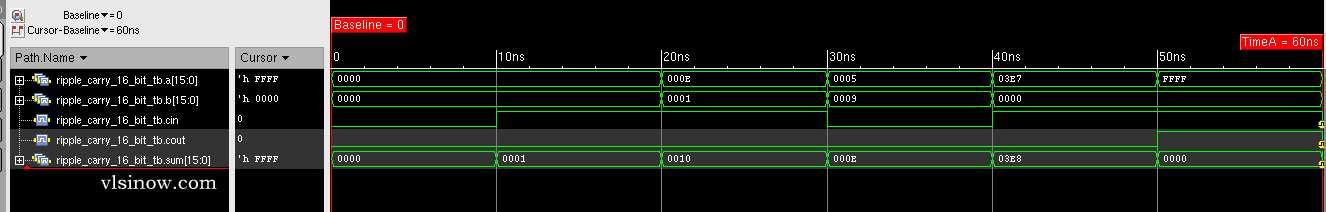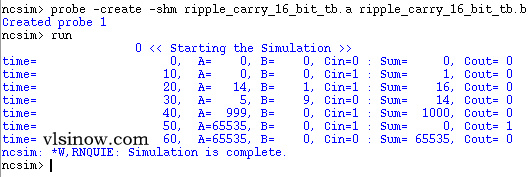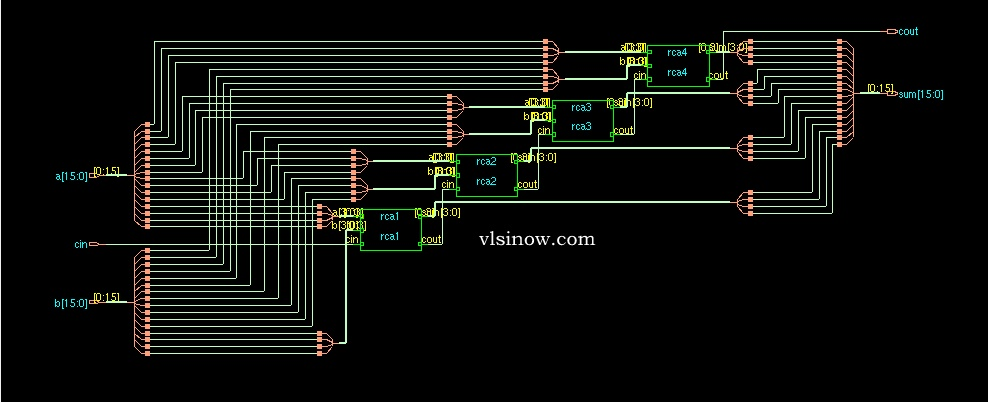# 16 bit Ripple Carry Adder Verilog Code

#### N- bit Ripple Carry Adder Verilog Code

Ripple carry adder is the basic adder architecture.  In ripple carry adder the carry bit may  ripple from first bit to last bit. Hence we call it  ‘Ripple Carry Adder’ . The general structure of 4-bit ripple carry adder is shown below.Here we can see the LSB bits are A0, B0 and C0 where C0 is the input carry bit.  Output of 1-bit full adder is S0 and C1.

where S0= LSB sum bit   and C1=Carry Out of first 1-bit full adder

The boolean equation for 1 bit full adder output, S0 and C1, is given  by

S0=A0⊕B0⊕C0  and   C1= (A0⊕B0).C0 + A0B0.

A 4-bit Ripple Carry adder is built by  cascading four 1-bit full adders in series.  The out  carry bit of every full adder is fed to the input of the next full adder.

Now as we have 4-bit ripple carry adder so it can further be cascaded in series to get higher bit adder.

For example, we can get 16 -bit Ripple Carry Adder by cascading in series four 4-bit ripple carry adders. While writing the Verilog code for 16-bit Ripple carry adder the same procedure is used. First the Verilog code for 1-bit full adder is written. From this, we can get the 4-bit ripple carry adder.  Now, by using  this 4-bit ripple carry adder  16-bit ripple carry adder Verilog code has been written.

Similar way, we can get N-bit ripple carry adder.

The Verilog Code for 16-bit Ripple  Carry is given below-

````timescale 1ns / 1ps
//////////////////////////////////////
/////////////////////////////////////

module ripple_carry_16_bit(a, b, cin,sum, cout);
input [15:0] a,b;
input cin;
output [15:0] sum;
output cout;
wire c1,c2,c3;

ripple_carry_4_bit rca1 (
.a(a[3:0]),
.b(b[3:0]),
.cin(cin),
.sum(sum[3:0]),
.cout(c1));

ripple_carry_4_bit rca2(
.a(a[7:4]),
.b(b[7:4]),
.cin(c1),
.sum(sum[7:4]),
.cout(c2));

ripple_carry_4_bit rca3(
.a(a[11:8]),
.b(b[11:8]),
.cin(c2),
.sum(sum[11:8]),
.cout(c3));

ripple_carry_4_bit rca4(
.a(a[15:12]),
.b(b[15:12]),
.cin(c3),
.sum(sum[15:12]),
.cout(cout));
endmodule

////////////////////////////////////
////////////////////////////////////

module ripple_carry_4_bit(a, b, cin, sum, cout);
input [3:0] a,b;
input cin;
wire c1,c2,c3;
output [3:0] sum;
output cout;

endmodule

//////////////////////////////
/////////////////////////////
input a,b,cin;
output sum, cout;
wire x,y,z;
or or_1(cout,z,y);
endmodule

///////////////////////////
//////////////////////////
module half_adder( a,b, sum, cout );
input a,b;
output sum, cout;
xor xor_1 (sum,a,b);
and and_1 (cout,a,b);
endmodule```

We are here providing the testbench for 16-bit Ripple Carry Adder

````timescale 1ns / 1ps
module ripple_carry_16_bit_tb;
wire [15:0] sum;//output
wire cout;//output
reg [15:0] a,b;//input
reg cin;//input

ripple_carry_16_bit uut(
.a(a),
.b(b),
.cin(cin),
.sum(sum),
.cout(cout));

initial begin
\$display(\$time, " << Starting the Simulation >>");
a=0; b=0; cin=0;
#100 a= 16'b0000000000011111; b=16'b000000000001100; cin=1'b0;
#10 a= 16'b0000000000011111; b=16'b000000000001100; cin=1'b0;
#10 a= 16'b1100011000011111; b=16'b000000110001100; cin=1'b1;
#10 a= 16'b1111111111111111; b=16'b000000000000000; cin=1'b1;
end

initial
\$monitor("time= ",
\$time,
"A=%b,
B=&b,
Cin=%b
: Sum= %b,
Cout=%cout",
a,b,cin,sum,cout);
endmodule```

16 bit Ripple Carry Adder Simulation Results:Also the monitor command result is –Cadence RTL compiler is used to synthesize the verilog  code with Uofu standard library.  We got the following schematic after mapping the hdl code to the standard library. This is basically the synthesized view.If you have any query/suggestion please feel free to comment below the post.View all posts by admin →